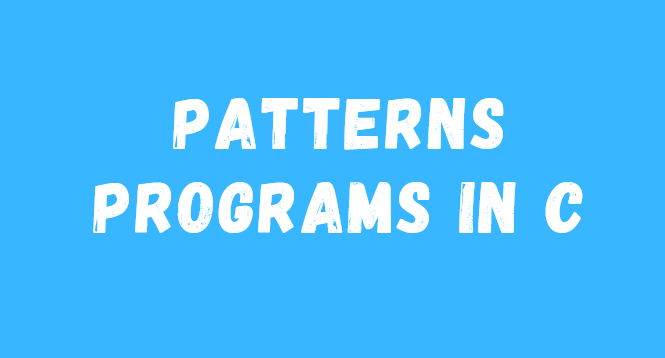Patterns Programs in C (With Popular Examples) - Do Tech Blog## What is Patterns Programs in C

Programs in which the output is shown as a pattern such as a star pattern or number and character in Patten.

The nested loops are used to create programs in this logical manner called pattern programs.

In this article, we will see many patterns programs as well as learn what logic is applied in these programs.

## Print Alphabet Patterns Programs in c

#include <stdio.h>

#include <conio.h>

void main ()

{

int a, b;

for (a = 1; a <= 5; a ++)

{

for (b = 1; b <= a; b ++)

{

printf ("% c", 'A' + b-1);

}

printf ("\ n");

}

getch ();

}

Output

A

AB

ABC

ABCD

ABCDE

Explanation

In the program, we have taken two variables a and b.

Then, with the help of a nested loop, for loop me a has a value of 1, and the condition of the loop is an increment until a <= 5 condition is true.

Put 1 value in b in the second for loop of the nested loop and the condition b increment of the loop until b <= a condition is true.

A value of b will be added when loop runs.

## Print Alphabet Patterns Programs in C

#include <stdio.h>

#include <conio.h>

void main ()

{

int a, b;

for (a = 1; a <= 5; a ++)

{

for (b = 1; b <= a; b ++)

{

printf ("% c", 'A'-1 + a);

}

printf ("\ n");

}

getch ();

}

Output

A

BB

CCC

DDDD

EEEEE

Explanation

In the program, we have taken two variables a and b

Then, with the help of nested loop, for loop me a has a value of 1 and the condition of the loop is an increment until a <= 5 condition is true

Put 1 value in b in the second for loop of the nested loop and the condition b increment of the loop until b <= a condition is true

Results will show using printf.

## Pattern Program in C

#include <stdio.h>

int main ()

{

int row, x, y;

printf ("Enter the zber of rows \ n");

scanf ("% d", & y);

for (row = 1; row <= y; row ++)

{

for (x = 1; x <= y-row; x ++)

printf ("");

for (x = 1; x <= 2 * row - 1; x ++)

printf ("*");

printf ("\ n");

}

return 0;

}

Input

Enter the number of rows 6

Output

*

**

***

****

*****

******

In the program, we have taken three integer variables x, y, and row

Then, with the help of nested loop, put 1 value in for loop row and the condition of the loop is row increment until row <= y condition is true

Two for loops of the nested loop are used

for (x = 1; x <= y-row; x ++)

&

for (x = 1; x <= 2 * row - 1; x ++)

And to show in result star patten * sign is printed

## Print Alphabet Pattern Program in C

#include <stdio.h>

#include <conio.h>

void main ()

{

int a, b;

for (a = 1; a <= 5; a ++)

{

for (b = 1; b <= a; b ++)

{

if (b% 2 == 0) printf ("A");

else printf ("*");

}

printf ("\ n");

}

getch ();

}

Output

*

* A *

* A * A *

* A * A * A *

#include <stdio.h>

#include <conio.h>

int main ()

{

int rows, space, disp, x, y, z = 1;

printf ("Enter number of rows \ n");

// less Than 12

scanf ("% d", & x);

y = x;

for (rows = 1; rows <= x; rows ++)

{

for (space = 1; space <= y; space ++) {

printf ("", y);

} y--;

for (disp = 1; disp <= rows; disp ++) {

if (z <10) {

printf ("% d", z);

}

else {

printf ("% d", z);

}

z ++;

}

printf ("\ n");

}

return 0;

}

Input

Enter the number of rows  6

Output

******

*****

****

***

Recommend: Call By Value And Call By Reference